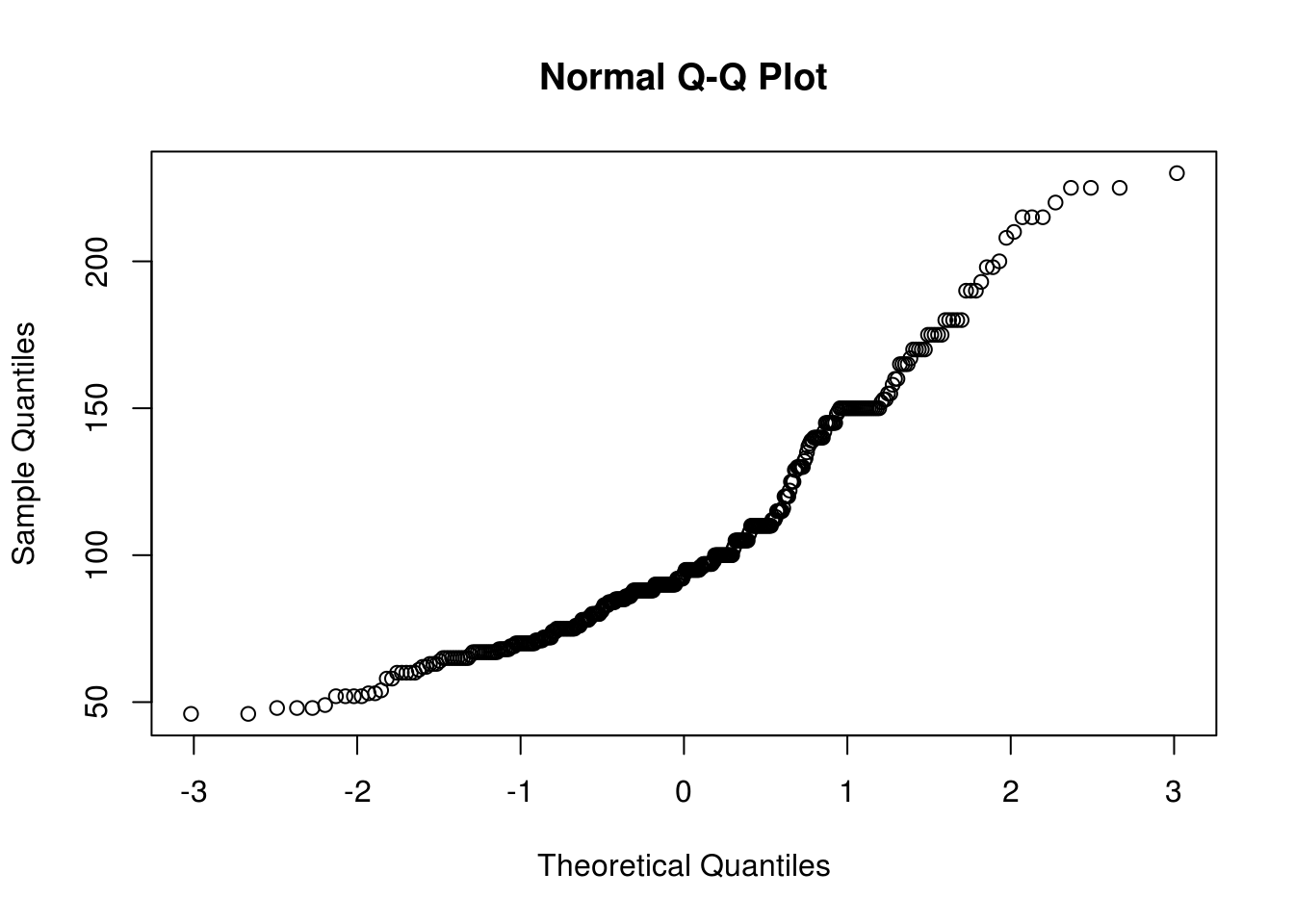# Theoretical Distribution and R

This post will explore an example of testing if a dataset fits a specific theoretical distribution. This is a very important aspect of statistical modeling as it allows to understand the normality of the data and the appropriate steps needed to take to prepare for analysis.

In our example, we will use the “Auto” dataset from the “ISLR” package. We will check if the horsepower of the cars in the dataset is normally distributed or not. Below is some initial code to begin the process.

``````library(ISLR)
library(nortest)
library(fBasics)``````
``data("Auto")``

Determining if a dataset is normally distributed is simple in R. This is normally done visually through making a Quantile-Quantile plot (Q-Q plot). It involves using two functions the “qnorm” and the “qqline”. Below is the code for the Q-Q plot

``qqnorm(Auto\$horsepower)``We now need to add the Q-Q line to see how are distribution lines up with the theoretical normal one. Below is the code. Note that we have to repeat the code above in order to get the completed plot.

``````qqnorm(Auto\$horsepower)
qqline(Auto\$horsepower, distribution = qnorm, probs=c(.25,.75))``````The “qqline” function needs the data you want to test as well as the distribution and probability. The distribution we wanted is normal and is indicated by the argument “qnorm”. The probs argument means probability. The default values are .25 and .75. The resulting graph indicates that the distribution of “horsepower”, in the “Auto” dataset is not normally distributed. That are particular problems with the lower and upper values.

We can confirm our suspicion by running a statistical test. The Anderson-Darling test from the “nortest” package will allow us to test whether our data is normally distributed or not. The code is below

``ad.test(Auto\$horsepower)``
``````##  Anderson-Darling normality test
##
## data:  Auto\$horsepower
## A = 12.675, p-value < 2.2e-16``````

From the results, we can conclude that the data is not normally distributed. This could mean that we may need to use non-parametric tools for statistical analysis.

We can further explore our distribution in terms of its skew and kurtosis. Skew measures how far to the left or right the data leans and kurtosis measures how peaked or flat the data is. This is done with the “fBasics” package and the functions “skewness” and “kurtosis”.

First we will deal with skewness. Below is the code for calculating skewness.

``````horsepowerSkew<-skewness(Auto\$horsepower)
horsepowerSkew``````
``````##  1.079019
## attr(,"method")
##  "moment"``````

We now need to determine if this value of skewness is significantly different from zero. This is done with a simple t-test. We must calculate the t-value before calculating the probability. The standard error of the skew is defined as the square root of six divided by the total number of samples. The code is below

``````stdErrorHorsepower<-horsepowerSkew/(sqrt(6/length(Auto\$horsepower)))
stdErrorHorsepower``````
``````##  8.721607
## attr(,"method")
##  "moment"``````

Now we take the standard error of Horsepower and plug this into the “pt” function (t probability) with the degrees of freedom (sample size – 1 = 391) we also put in the number 1 and subtract all of this information. Below is the code

``1-pt(stdErrorHorsepower,391)``
``````##  0
## attr(,"method")
##  "moment"``````

The value zero means that we reject the null hypothesis that the skew is not significantly different form zero and conclude that the skew is different form zero. However, the value of the skew was only 1.1 which is not that non-normal.

We will now repeat this process for the kurtosis. The only difference is that instead of taking the square root divided by six we divided by 24 in the example below.

``````horsepowerKurt<-kurtosis(Auto\$horsepower)
horsepowerKurt``````
``````##  0.6541069
## attr(,"method")
##  "excess"``````
``````stdErrorHorsepowerKurt<-horsepowerKurt/(sqrt(24/length(Auto\$horsepower)))
stdErrorHorsepowerKurt``````
``````##  2.643542
## attr(,"method")
##  "excess"``````
``1-pt(stdErrorHorsepowerKurt,391)``
``````##  0.004267199
## attr(,"method")
##  "excess"``````

Again the pvalue is essentially zero, which means that the kurtosis is significantly different from zero. With a value of 2.64 this is not that bad. However, when both skew and kurtosis are non-normally it explains why our overall distributions was not normal either.

Conclusion

This post provided insights into assessing the normality of a dataset. Visually inspection can take place using  Q-Q plots. Statistical inspection can be done through hypothesis testing along with checking skew and kurtosis.